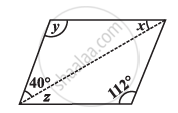Share

# Consider the Given Parallelograms. Find the Values of the Unknowns X, Y, Z. - 5 - CBSE Class 8 - Mathematics

ConceptKinds of Quadrilaterals Concept of Parallelogram

#### Question

Consider the given parallelograms. Find the values of the unknowns x, y, z.#### Solution

y = 112° (Opposite angles are equal)

x+ y + 40° = 180° (Angle sum property of triangles)

+ 112° + 40° = 180°

+ 152° = 180°

x = 28°

z = x = 28° (Alternate interior angles)

Is there an error in this question or solution?

#### APPEARS IN

NCERT Solution for Mathematics Textbook for Class 8 (2018 to Current)